# 14-240/Tutorial-October28

## Boris

#### Be Efficient

By this point in the course, we become good at solving systems of linear equations. However, we should not use this same old problem-solving strategy over and over if a more efficient one exists. Consider the following problems:

Q1: Determine if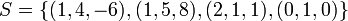$S = \{(1, 4, -6), (1, 5, 8), (2, 1, 1), (0, 1, 0)\}$ is linearly independent in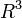$R^3$.

We can solve this linear equation to find the answer: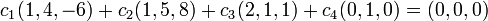$c_1(1, 4, -6) + c_2(1, 5, 8) + c_3 (2, 1, 1) + c_4(0, 1, 0) = (0, 0, 0)$ where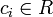$c_i \in R$.

Yet there is a less time-consuming approach that relies on two observations:

(1) The dimension of$R^3$ is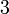$3$ so the size of a basis is also$3$.
(2) No linearly independent set can have more vectors than a generating set (by the Replacement Theorem).

Since a basis is a generating set and the size of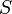$S$ is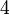$4$, then the Replacement Theorem tells us that$S$ cannot be linearly independent. Hence, the problem can be solved without solving any linear equations.

Q2: Determine if the polynomials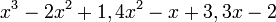$x^3 - 2x ^2 + 1, 4x^2-x+3, 3x-2$ generate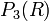$P_3(R)$.

Once again, we can solve a linear equation but we do not have to. Observe:

(1) The dimension$P_3(R)$ is$4$ so the size of a basis is also$4$.
(2) No generating set can have fewer vectors than a basis (by a Corollary to the Replacement Theorem).

Since there are only$3$ polynomials, then the Corollary tells us that it cannot generate$P_3(R)$. Once again, we used a more efficient strategy.

#### Extending a Linearly Independent Set to a Basis

Boris's tip (for concrete sets and vector spaces only):

If a problem requires us to extend a linearly independent set to a basis, then the easiest approach is to add vectors from the standard ordered basis. Here is an example:

Let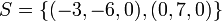$S = \{(-3, -6, 0), (0, 7, 0)\}$ be a linearly independent subset of$R^3$. To extend$S$ to a basis, add vectors from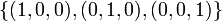$\{(1, 0, 0), (0, 1, 0), (0, 0, 1)\}$. The only question is which vector(s) should we add?

We see that both vectors in$S$ have a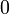$0$ as the third component so a safe choice is to add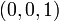$(0, 0, 1)$. Since$R^3$ has a dimension of$3$, then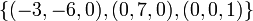$\{(-3, -6, 0), (0, 7, 0), (0, 0, 1)\}$ is a basis of$R^3$.